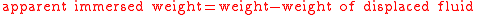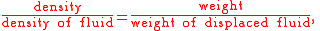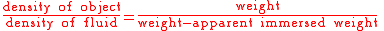xArchimedes' principleEncyclopedia
Archimedes' principle relates buoyancy
Buoyancy
In physics, buoyancy is a force exerted by a fluid that opposes an object's weight. In a column of fluid, pressure increases with depth as a result of the weight of the overlying fluid. Thus a column of fluid, or an object submerged in the fluid, experiences greater pressure at the bottom of the...

to displacement
Displacement (fluid)
In fluid mechanics, displacement occurs when an object is immersed in a fluid, pushing it out of the way and taking its place. The volume of the fluid displaced can then be measured, as in the illustration, and from this the volume of the immersed object can be deduced .An object that sinks...

. It is named after its discoverer, Archimedes of Syracuse
Archimedes
Archimedes of Syracuse was a Greek mathematician, physicist, engineer, inventor, and astronomer. Although few details of his life are known, he is regarded as one of the leading scientists in classical antiquity. Among his advances in physics are the foundations of hydrostatics, statics and an...

.

## Principle

Archimedes' treatise On floating bodies, proposition 5, states that
For more general objects, floating and sunken, and in gasses as well as liquids (i.e. a fluid
Fluid
In physics, a fluid is a substance that continually deforms under an applied shear stress. Fluids are a subset of the phases of matter and include liquids, gases, plasmas and, to some extent, plastic solids....

), Archimedes' principle may be stated thus in terms of forces:
with the clarifications that for a sunken object the volume of displaced fluid is the volume of the object, and for a floating object on a liquid, the weight of the displaced liquid is the weight of the object.

In short, buoyancy = weight of displaced fluid.

## Refinements

Archimedes' principle does not consider the surface tension
Surface tension
Surface tension is a property of the surface of a liquid that allows it to resist an external force. It is revealed, for example, in floating of some objects on the surface of water, even though they are denser than water, and in the ability of some insects to run on the water surface...

(capillarity) acting on the body.

## Formula

The weight of the displaced fluid is directly proportional to the volume of the displaced fluid (if the surrounding fluid is of uniform density). In simple terms, the principle states that the buoyant force on an object is going to be equal to the weight of the fluid displaced by the object, or the density of the fluid multiplied by the submerged volume times the gravitational constant, g. Thus, among completely submerged objects with equal masses, objects with greater volume have greater buoyancy.

Suppose a rock's weight is measured as 10 newtons when suspended by a string in a vacuum
Vacuum
In everyday usage, vacuum is a volume of space that is essentially empty of matter, such that its gaseous pressure is much less than atmospheric pressure. The word comes from the Latin term for "empty". A perfect vacuum would be one with no particles in it at all, which is impossible to achieve in...

with gravity acting upon it. Suppose that when the rock is lowered into water, it displaces water of weight 3 newtons. The force it then exerts on the string from which it hangs would be 10 newtons minus the 3 newtons of buoyant force: 10 − 3 = 7 newtons. Buoyancy reduces the apparent weight of objects that have sunk completely to the sea floor. It is generally easier to lift an object up through the water than it is to pull it out of the water.

Assuming Archimedes' principle to be reformulated as follows,then inserted into the quotient of weights, which has been expanded by the mutual volumeyields the formula below. The density of the immersed object relative to the density of the fluid can easily be calculated without measuring any volumes:(This formula is used for example in describing the measuring principle of a dasymeter
Dasymeter
A dasymeter was meant initially as a device to demonstrate the buoyant effect of gases like air; as shown in the pictures on the right. A dasymeter which allows weighing acts as a densimeter used to measure the density of gases.-Principle:...

and of hydrostatic weighing
Hydrostatic weighing
Hydrostatic weighing is a method for measuring the mass density of a body.-Method:The following method has the advantage of needing no volume information of the body...

.)

Example: If you drop wood into water buoyancy will keep it afloat.

Example: A helium balloon in a moving car. In increasing speed or driving a curve, the air moves in the opposite direction of the car's acceleration. The balloon however, is pushed due to buoyancy "out of the way" by the air, and will actually drift in the same direction as the car's acceleration.